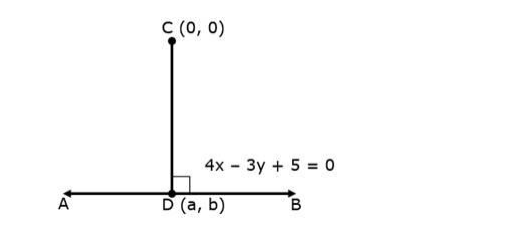# Find the equation of the perpendicular drawn from the origin to the line`
Question:

Find the equation of the perpendicular drawn from the origin to the line 4x – 3y + 5 = 0. Also, find the coordinates of the foot of the perpendicular.

Solution:Let the equation of line $A B$ be $4 x-3 y+5=0$

and point C be (0, 0)

CD is perpendicular to the line AB, and we need to find:

1) Equation of Perpendicular drawn from point $C$

2) Coordinates of $D$

Let the coordinates of point D be (a, b)

Also, point D(a, b) lies on the line AB, i.e. point (a, b) satisfy the equation of line AB

Putting x = a and y = b, in equation, we get

$4 a-3 b+5=0 \ldots$ (i)

Also, the CD is perpendicular to the line AB

and we know that, if two lines are perpendicular then the product of their slope is equal to -1

$\therefore$ Slope of $\mathrm{AB} \times$ Slope of $\mathrm{CD}=-1$

$\Rightarrow$ Slope of $\mathrm{CD}=\frac{-1}{\text { Slope of } \mathrm{AB}}$

$=\frac{-1}{\frac{4}{3}}$

Slope of CD $=-\frac{3}{4}$

Now, Equation of line $C D$ formed by joining the points $C(0,0)$ and $D(a, b)$ and having the slope $-\frac{3}{4}$ is

$\mathrm{y}_{2}-\mathrm{y}_{1}=\mathrm{m}\left(\mathrm{x}_{2}-\mathrm{x}_{1}\right)$

$\Rightarrow b-0=-\frac{3}{4}(a-0)$

$\Rightarrow \mathrm{b}=-\frac{3}{4} \mathrm{a}$

$\Rightarrow 4 b=-3 a$

$\Rightarrow 3 a+4 b=0 \ldots$ (ii)

Now, our equations are

$4 a-3 b+5=0 \ldots$ (i)

and $3 a+4 b=0 \ldots$ (ii)

Multiply the eq. (i) by 4 and (ii) by 3, we get

$16 a-12 b+20=0 \ldots$ (iii)

$9 a+12 b=0 \ldots$ (iv)

Adding eq. (iii) and (iv), we get

$16 a-12 b+20+9 a+12 b=0$

$\Rightarrow 25 a+20=0$

$\Rightarrow 25 a=-20$

$\Rightarrow \mathrm{a}=-\frac{20}{25}=-\frac{4}{5}$

Putting the value of a in eq. (ii), we get

$3\left(-\frac{4}{5}\right)+4 b=0$

$\Rightarrow-\frac{12}{5}+4 \mathrm{~b}=0$

$\Rightarrow-12+20 b=0$

$\Rightarrow 20 b=12$

$\Rightarrow \mathrm{b}=\frac{12}{20}$

$\Rightarrow \mathrm{b}=\frac{3}{5}$

Hence, the coordinates of $\mathrm{D}(\mathrm{a}, \mathrm{b})$ is $\left(-\frac{4}{5}, \frac{3}{5}\right)$